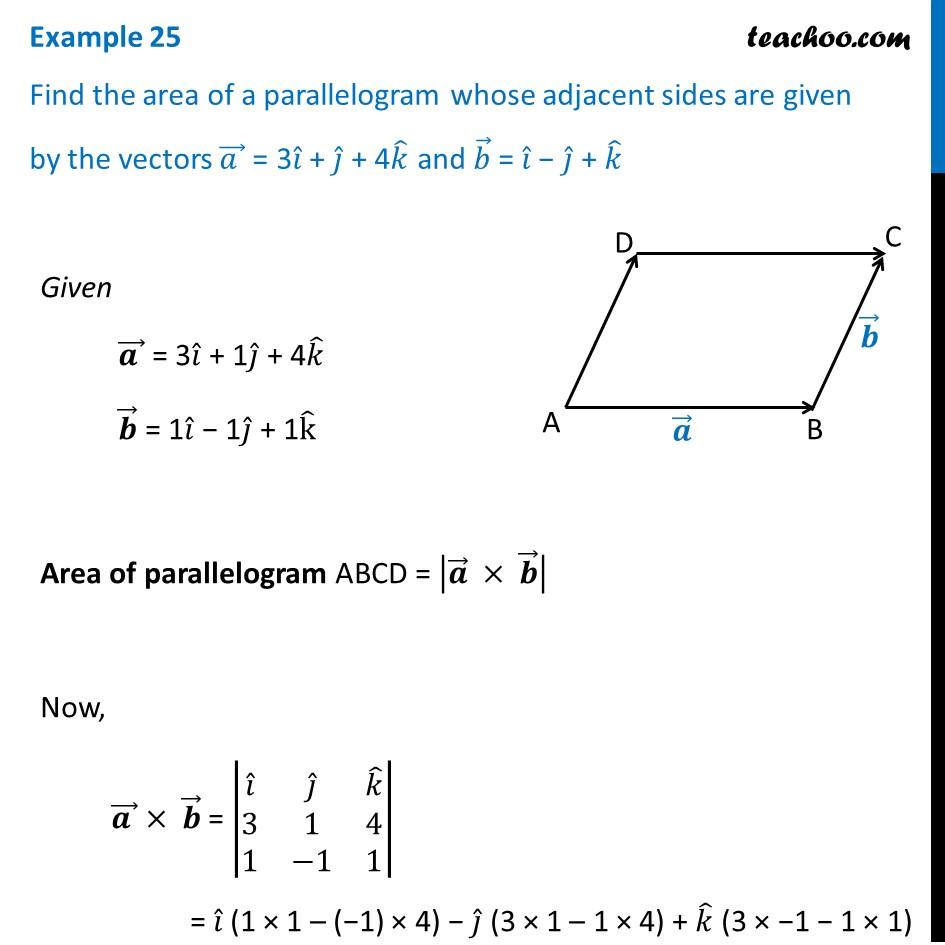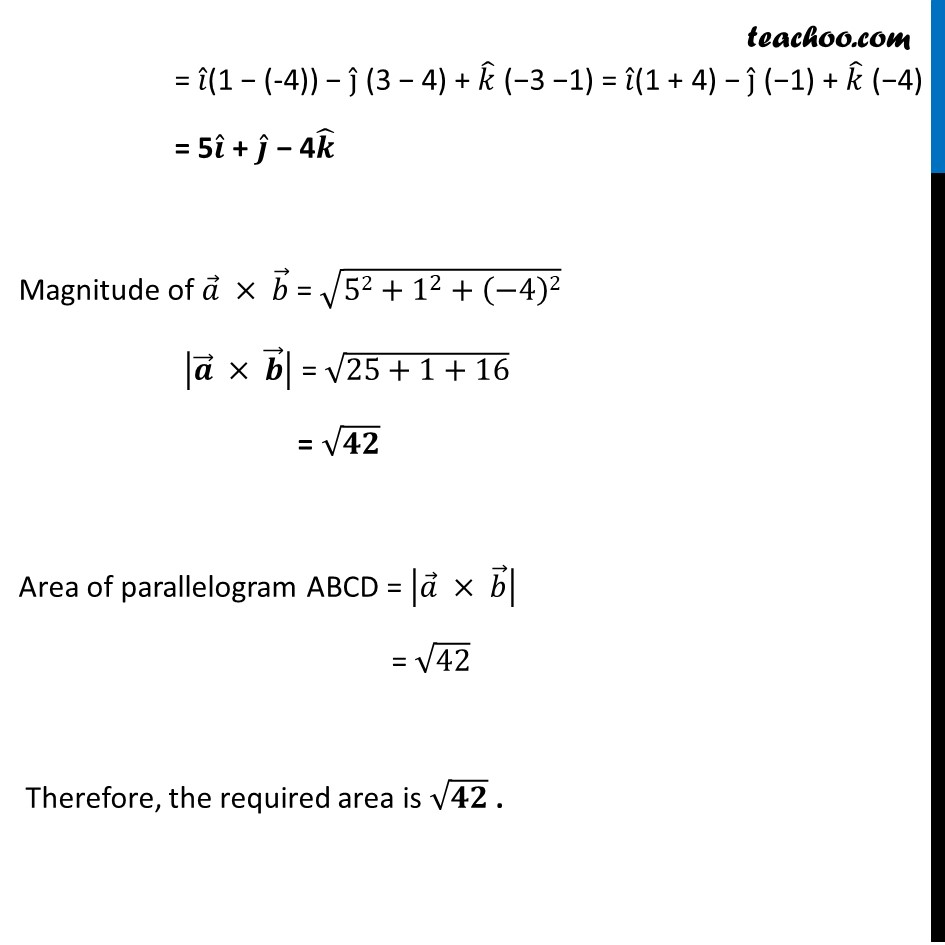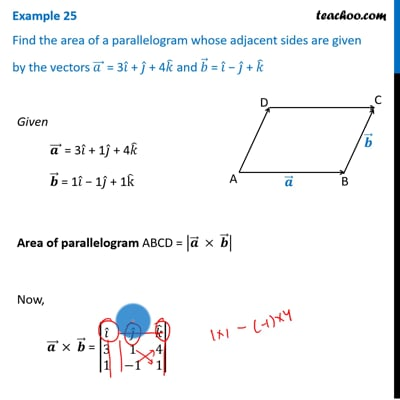Vector product - Area

Chapter 10 Class 12 Vector Algebra
Concept wiseThis video is only available for Teachoo black users

Introducing your new favourite teacher - Teachoo Black, at only ₹83 per month

### Transcript

Example 25 Find the area of a parallelogram whose adjacent sides are given by the vectors (𝑎 ) ⃗ = 3𝑖 ̂ + 𝑗 ̂ + 4𝑘 ̂ and 𝑏 ⃗ = 𝑖 ̂ − 𝑗 ̂ + 𝑘 ̂ Given (𝒂 ) ⃗ = 3𝑖 ̂ + 1𝑗 ̂ + 4𝑘 ̂ 𝒃 ⃗ = 1𝑖 ̂ − 1𝑗 ̂ + 1k ̂ Area of parallelogram ABCD = |𝒂 ⃗ × 𝒃 ⃗ | Now, (𝒂 ) ⃗× 𝒃 ⃗ = |■8(𝑖 ̂&𝑗 ̂&𝑘 ̂@3&1&4@1&−1&1)| = 𝑖 ̂ (1 × 1 – (−1) × 4) − 𝑗 ̂ (3 × 1 – 1 × 4) + 𝑘 ̂ (3 × −1 − 1 × 1) = 𝑖 ̂(1 − (-4)) − j ̂ (3 − 4) + 𝑘 ̂ (−3 −1) = 𝑖 ̂(1 + 4) − j ̂ (−1) + 𝑘 ̂ (−4) = 5𝒊 ̂ + 𝒋 ̂ − 4𝒌 ̂ Magnitude of 𝑎 ⃗ × 𝑏 ⃗ = √(52+1^2+(−4)2) |𝒂 ⃗ × 𝒃 ⃗ | = √(25+1+16) = √𝟒𝟐 Area of parallelogram ABCD = |𝑎 ⃗ × 𝑏 ⃗ | = √42 Therefore, the required area is √𝟒𝟐 .